## Programming Assignment 5: Kd-Trees

Create a symbol table data type whose keys are two-dimensional points. Use a 2d-tree to support efficient range search (find all of the points contained in a query rectangle) and nearest neighbor search (find a closest point to a query point). 2d-trees have numerous applications, ranging from classifying astronomical objects to computer animation to speeding up neural networks to mining data to image retrieval.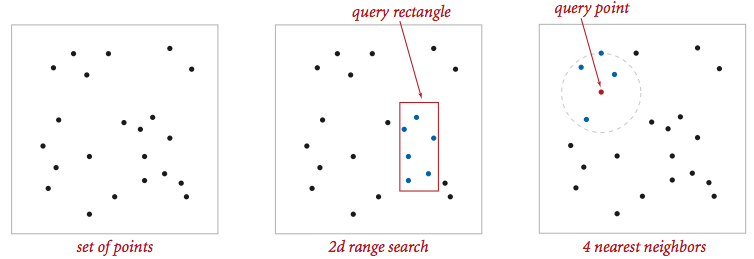Geometric primitives. To get started, use the following geometric primitives for points and axis-aligned rectangles in the plane.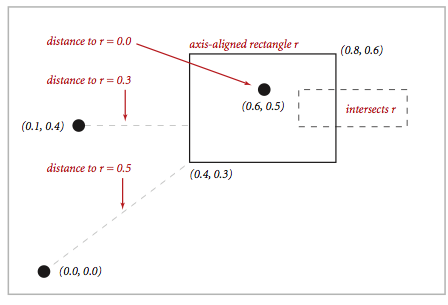Use the immutable data type Point2D (part of algs4.jar) for points in the plane. Here is the subset of its API that you may use:

```public class Point2D implements Comparable<Point2D> {
public Point2D(double x, double y)              // construct the point (x, y)
public  double x()                              // x-coordinate
public  double y()                              // y-coordinate
public  double distanceSquaredTo(Point2D that)  // square of Euclidean distance between two points
public     int compareTo(Point2D that)          // for use in an ordered symbol table
public boolean equals(Object that)              // does this point equal that object?
public  String toString()                       // string representation
}
```
Use the immutable data type RectHV (part of algs4.jar) for axis-aligned rectanges. Here is the subset of its API that you may use:
```public class RectHV {
public    RectHV(double xmin, double ymin,      // construct the rectangle [xmin, xmax] x [ymin, ymax]
double xmax, double ymax)
public  double xmin()                           // minimum x-coordinate of rectangle
public  double ymin()                           // minimum y-coordinate of rectangle
public  double xmax()                           // maximum x-coordinate of rectangle
public  double ymax()                           // maximum y-coordinate of rectangle
public boolean contains(Point2D p)              // does this rectangle contain the point p (either inside or on boundary)?
public boolean intersects(RectHV that)          // does this rectangle intersect that rectangle (at one or more points)?
public  double distanceSquaredTo(Point2D p)     // square of Euclidean distance from point p to closest point in rectangle
public boolean equals(Object that)              // does this rectangle equal that object?
public  String toString()                       // string representation
}
```
Do not modify these data types.

Brute-force implementation. Write a mutable data type PointST.java that is symbol table with Point2D. Implement the following API by using a red-black BST (using either RedBlackBST from algs4.jar or java.util.TreeMap); do not implement your own red-black BST.

```public class PointST<Value> {
public         PointST()                                // construct an empty symbol table of points
public           boolean isEmpty()                      // is the symbol table empty?
public               int size()                         // number of points
public              void put(Point2D p, Value val)      // associate the value val with point p
public             Value get(Point2D p)                 // value associated with point p
public           boolean contains(Point2D p)            // does the symbol table contain point p?
public Iterable<Point2D> points()                       // all points in the symbol table
public Iterable<Point2D> range(RectHV rect)             // all points that are inside the rectangle
public           Point2D nearest(Point2D p)             // a nearest neighbor to point p; null if the symbol table is empty
public static void main(String[] args)                  // unit testing (required)
}
```
Corner cases.  Throw a java.lang.NullPointerException if any argument is null. Performance requirements.  Your implementation should support size() in constant time; it should support put(), get() and contains() in time proportional to the logarithm of the number of points in the set in the worst case; and it should support points(), nearest(), and range() in time proportional to the number of points in the symbol table.

2d-tree implementation. Write a mutable data type KdTreeST.java that uses a 2d-tree to implement the same API (but renaming PointST to KdTreeST). A 2d-tree is a generalization of a BST to two-dimensional keys. The idea is to build a BST with points in the nodes, using the x- and y-coordinates of the points as keys in strictly alternating sequence, starting with the x-coordinates.

• Search and insert. The algorithms for search and insert are similar to those for BSTs, but at the root we use the x-coordinate (if the point to be inserted has a smaller x-coordinate than the point at the root, go left; otherwise go right); then at the next level, we use the y-coordinate (if the point to be inserted has a smaller y-coordinate than the point in the node, go left; otherwise go right); then at the next level the x-coordinate, and so forth.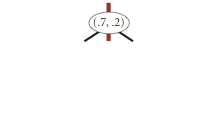insert (0.7, 0.2)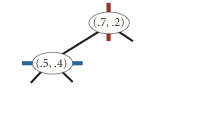insert (0.5, 0.4)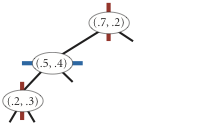insert (0.2, 0.3)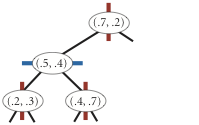insert (0.4, 0.7)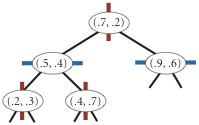insert (0.9, 0.6)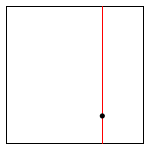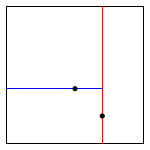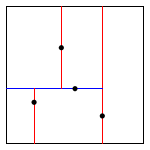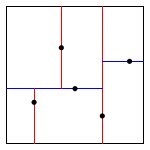• Level-order traversal. The points() method should return the points in level-order: first the root, then all children of the root (from left/bottom to right/top), then all grandchildren of the root (from left to right), and so forth. The level-order traversal of the 2d-tree above is (0.7, 0.2), (0.5, 0.4), (0.9, 0.6), (0.2, 0.3), (0.4, 0.7). This method is mostly useful to assist you (when debugging) and us (when grading).

The prime advantage of a 2d-tree over a BST is that it supports efficient implementation of range search and nearest neighbor search. Each node corresponds to an axis-aligned rectangle, which encloses all of the points in its subtree. The root corresponds to the infinitely large square from [(-∞, -∞), (+∞, +∞ )]; the left and right children of the root correspond to the two rectangles split by the x-coordinate of the point at the root; and so forth.

• Range search. To find all points contained in a given query rectangle, start at the root and recursively search for points in both subtrees using the following pruning rule: if the query rectangle does not intersect the rectangle corresponding to a node, there is no need to explore that node (or its subtrees). That is, you should search a subtree only if it might contain a point contained in the query rectangle.

• Nearest neighbor search. To find a closest point to a given query point, start at the root and recursively search in both subtrees using the following pruning rule: if the closest point discovered so far is closer than the distance between the query point and the rectangle corresponding to a node, there is no need to explore that node (or its subtrees). That is, you should search a node only if it might contain a point that is closer than the best one found so far. The effectiveness of the pruning rule depends on quickly finding a nearby point. To do this, organize your recursive method so that when there are two possible subtrees to go down, you choose first the subtree that is on the same side of the splitting line as the query point; the closest point found while exploring the first subtree may enable pruning of the second subtree.

Clients.  You may use the following two interactive client programs to test and debug your code.

• RangeSearchVisualizer.java reads a sequence of points from a file (specified as a command-line argument) and inserts those points into a 2d-tree. Then, it performs range searches on the axis-aligned rectangles dragged by the user in the standard drawing window.

• NearestNeighborVisualizer.java reads a sequence of points from a file (specified as a command-line argument) and inserts those points into a 2d-tree. Then, it performs nearest neighbor queries on the point corresponding to the location of the mouse in the standard drawing window.

Analysis of running time and memory usage. Analyze the effectiveness of your approach to this problem by giving estimates of its time and space requirements.

• Give the total memory usage in bytes (using tilde notation) of your 2d-tree data structure as a function of the number of points N, using the memory-cost model from lecture and Section 1.4 of the textbook. Count all memory that is used by your 2d-tree, including memory for the nodes, points, and rectangles.

• How many nearest neighbor calculations can your 2d-tree implementation perform per second for input100K.txt (100,000 points) and input1M.txt (1 million points), where the query points are uniformly random points in the unit square? (Do not count the time to read in the points or to build the 2d-tree.) Repeat this question but with the brute-force implementation.

Challenge for the bored.  Add the following method to KdTreeST.java:

```public Iterable<Point2D> nearest(Point2D p, int k)
```
This method should return the k points that are closest to the query point (in any order); return all N points in the data structure if Nk. It should do this in an efficient manner, i.e. using the technique from kd-tree nearest neighbor search, not from brute force. Once you've completed this class, you'll be able to run BoidSimulator.java (which depends upon both Boid.java and Hawk.java). Behold their flocking majesty.

Submission.  Submit only PointST.java and KdTreeST.java. We will supply algs4.jar. Your may not call library functions except those in those in java.lang, java.util, and algs4.jar. Finally, submit a readme.txt file and answer the questions.

This assignment was developed by Kevin Wayne, with boid simulation by Josh Hug.